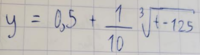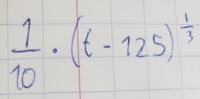# I didn't manage to differentiate this

#### Subhotosh Khan

##### Super Moderator
Staff member
Hi,
I have stumbled upon a little equation: I can't differentiate it.
Could anyon help me?
View attachment 28178
It's this one. I know how to deal with the products, and transform the root in fractional exponents, but I can't separate the t from the rest of the expression.
Air conditioner
It is not necessary to "separate" any variable to differentiate. Is the 't' independent variable? What is the dependent variable? "What" you have posted is not an equation - it does NOT have "equal" to ( = ) sign.

Why do you want to differentiate this expression - w.r.t. which variable?

Please post the EXACT problem as it was given to you.

Please show us what you have tried and exactly where you are stuck.

Please follow the rules of posting in this forum, as enunciated at:

#### Dr.Peterson

##### Elite Member
Hi,
I have stumbled upon a little equation: I can't differentiate it.
Could anyon help me?
View attachment 28178
It's this one. I know how to deal with the products, and transform the root in fractional exponents, but I can't separate the t from the rest of the expression.
Air conditioner
This is a function of t, so presumably you want to differentiate it with respect to t. I don't know what you mean by "separate the t from the rest of the expression".

Please show whatever you have tried, including "transform the root into fractional exponents", so we can help with the next step.

#### Air conditionner

##### New member
Well, this is the exercise:I want to find the derivative of y with respect to t. I know that 0.5 disappears since it's a constant. And after that, I can get this:But I can't go further... I don't know how to expand with fractional exponents. Should I use the binomial theorem? I used an application (Gauthmath) to try to get an answer, and they did give a solution (where they didn't use the binomial theorem) but I didn't understand the steps...

#### Dr.Peterson

##### Elite Member
Well, this is the exercise: View attachment 28179
I want to find the derivative of y with respect to t. I know that 0.5 disappears since it's a constant. And after that, I can get this:
View attachment 28180
But I can't go further... I don't know how to expand with fractional exponents. Should I use the binomial theorem? I used an application (Gauthmath) to try to get an answer, and they did give a solution (where they didn't use the binomial theorem) but I didn't understand the steps...
Do you know the chain rule? If u = t - 125, then this is 1/10 u^{1/3}, which you should be able to differentiate.

#### pka

##### Elite Member
If $$r\ne 0$$ is a real number then the derivative of $$(t-125)^r$$ is $$r(t-125)^{r-1}$$

#### Air conditionner

##### New member
Thank you very much.
I am gonna search and learn the chain rule!

#### Subhotosh Khan

##### Super Moderator
Staff member
Well, this is the exercise: View attachment 28179
I want to find the derivative of y with respect to t. I know that 0.5 disappears since it's a constant. And after that, I can get this:
View attachment 28180
But I can't go further... I don't know how to expand with fractional exponents. Should I use the binomial theorem? I used an application (Gauthmath) to try to get an answer, and they did give a solution (where they didn't use the binomial theorem) but I didn't understand the steps...
$$\displaystyle y \ = \ 0.5 \ + \ \sqrt{t \ - \ 125}$$.............. substitute $$\displaystyle u \ = \ -125 \ + \ t$$.........→...... $$\displaystyle \frac{du}{dt} \ = 1$$

$$\displaystyle y \ = \ 0.5 \ + \ \sqrt{u} \ = \ 0.5 + u^{\frac{1}{3}}$$

$$\displaystyle \frac{dy}{dt} \ = \frac{dy}{du} \ * \ \frac{du}{dt}$$ .................. apply chain rule

$$\displaystyle \frac{dy}{dt} \ = \left[ \frac{1}{3}u^{(\frac{1}{3}-1)}\right] \ * \ \frac{du}{dt}$$

$$\displaystyle \frac{dy}{dt} \ = \left[ \frac{1}{3}u^{(-\frac{2}{3})}\right] \ * \ (1)$$

$$\displaystyle \frac{dy}{dt} \ = \ \frac{1}{3 \ * u^{(\frac{2}{3})}}$$ ............. Now substitute back for "u".

#### Air conditionner

##### New member
$$\displaystyle y \ = \ 0.5 \ + \ \sqrt{t \ - \ 125}$$.............. substitute $$\displaystyle u \ = \ -125 \ + \ t$$.........→...... $$\displaystyle \frac{du}{dt} \ = 1$$

$$\displaystyle y \ = \ 0.5 \ + \ \sqrt{u} \ = \ 0.5 + u^{\frac{1}{3}}$$

$$\displaystyle \frac{dy}{dt} \ = \frac{dy}{du} \ * \ \frac{du}{dt}$$ .................. apply chain rule

$$\displaystyle \frac{dy}{dt} \ = \left[ \frac{1}{3}u^{(\frac{1}{3}-1)}\right] \ * \ \frac{du}{dt}$$

$$\displaystyle \frac{dy}{dt} \ = \left[ \frac{1}{3}u^{(-\frac{2}{3})}\right] \ * \ (1)$$

$$\displaystyle \frac{dy}{dt} \ = \ \frac{1}{3 \ * u^{(\frac{2}{3})}}$$ ............. Now substitute back for "u".
Thank you.